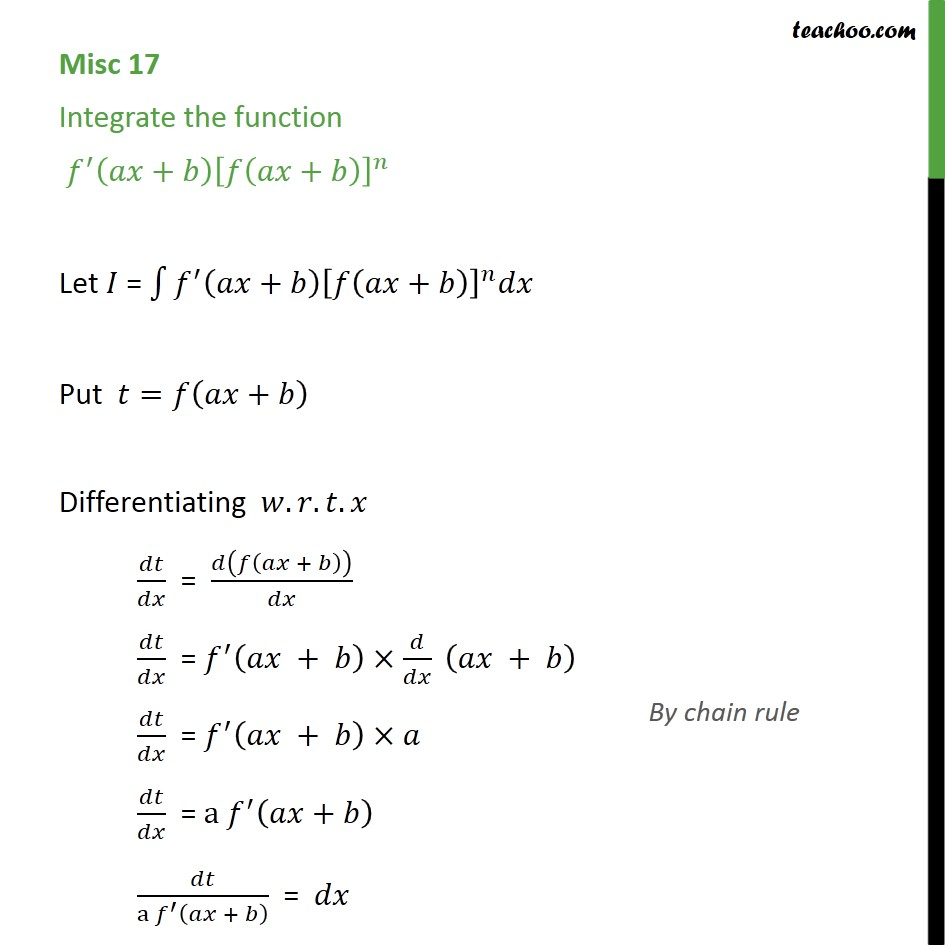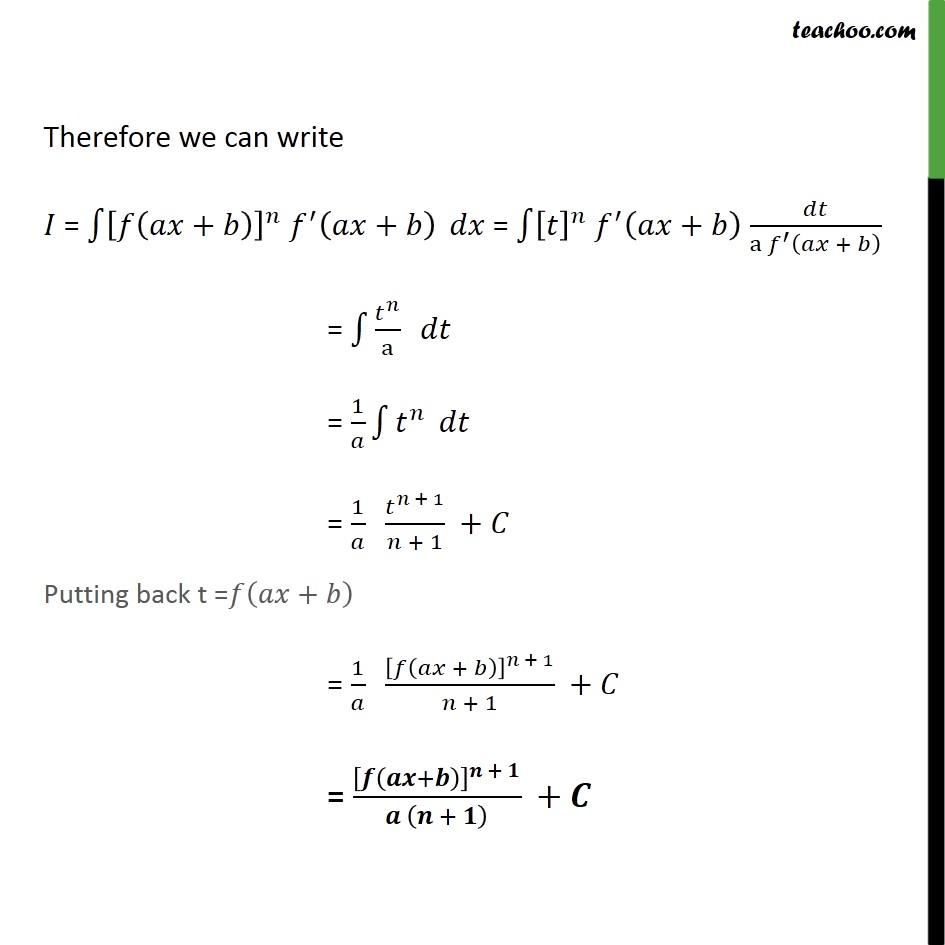Miscellaneous

Chapter 7 Class 12 Integrals
Serial order wiseLearn in your speed, with individual attention - Teachoo Maths 1-on-1 Class

### Transcript

Misc 17 Integrate the function + + Let = + + Put = + Differentiating . . . = + = + + = + = a + a + = Therefore we can write = + + = + a + = a = 1 = 1 + 1 + 1 + Putting back t = + = 1 + + 1 + 1 + = + + + +